edHelper subscribers - Create a new printable
Answer key also includes questions
Answer key only gives the answers
No answer key

Not a subscriber?  Sign up now for the subscriber materials!
Sample edHelper.com - Algebra Worksheet
Return to Trigonometry
Return to Math

 Name _____________________________Date ___________________
Sine
(Answer ID # 0297692)
Complete. Round to the nearest hundredth.

1.   Find sin M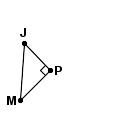MP
=  10.5PJ
=  9.25MJ
=  13.99
2.   Find sin D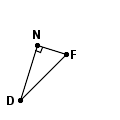DF
=  10.91DN
=  9.7FN
=  5
3.   Find sin S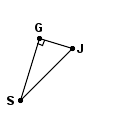SG
=  7.2JG
=  3.8SJ
=  8.14
4.   Find sin F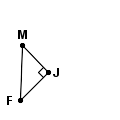FM
=  6.04FJ
=  4.43JM
=  4.1
5.   Find sin V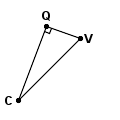CQ
=  11.3VQ
=  5.1CV
=  12.4
6.   Find sin T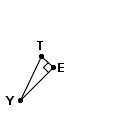ET
=  3.2YT
=  9.93YE
=  9.4
7.   Find sin B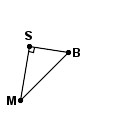MB
=  5.66MS
=  4.6BS
=  3.3
8.   Find sin B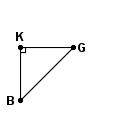BK
=  4.1GK
=  4.1BG
=  5.8
9.   Find sin B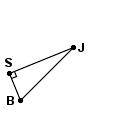BS
=  5.8JS
=  13.8BJ
=  14.97

 Answer Key

Sample
This is only a sample worksheet.

edHelper subscribers - Create a new printable
Answer key also includes questions
Answer key only gives the answers
No answer key

Not a subscriber?  Sign up now for the subscriber materials!
Sample edHelper.com - Algebra Worksheet
Return to Trigonometry
Return to Math